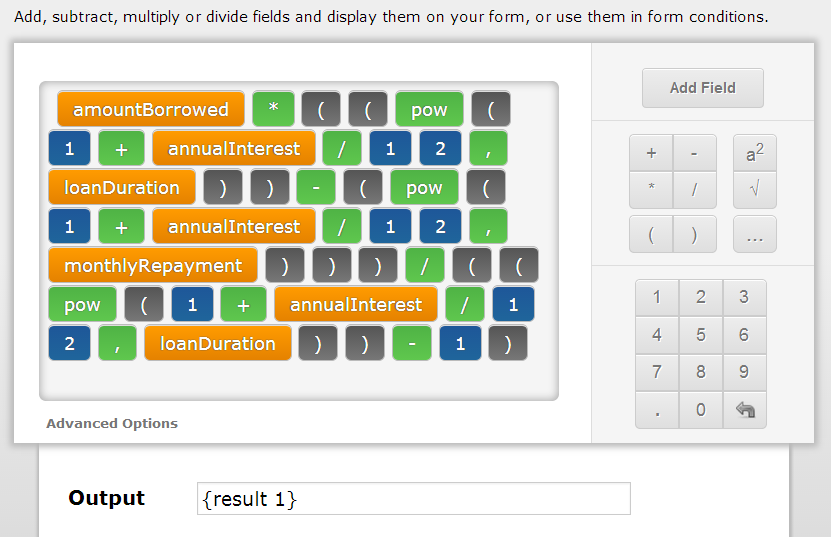# Mortgage calculator with remaining balance of the loan after a year

•htedeland
Asked on May 11, 2014 at 09:18 AM
Hi I am using Nicolas's template above to build my own mortgage calculator and am wondering if someone can help me add a field that calculates the remaining balance of the loan after 1 year?  I know the formula to calculate that but am unsure how to enter that in jotform (specifically, how to raise a number to a specific power)? thanks
•Welvin
Answered on May 11, 2014 at 09:35 AM

If possible, let us know the formula so we can check and try to incorporate it to the current calculation. I think this involves additional field for paid months or years, then less it to the loan duration.

Thanks

•htedeland
Answered on May 11, 2014 at 09:54 AM

Hi it is the second formula listed on this page:

http://www.mtgprofessor.com/formulas.htm

•BDAVID
Answered on May 11, 2014 at 01:53 PM

In order to raise a number to a specific power you need to use he "pow" function:

pow
Power, returns the value of x to the power of y. Example pow(2,3) which means 2*2*2, returns 8.
Example Formula:So, following the formula (B = L[(1 + c)n - (1 + c)p]/[(1 + c)n - 1]) , and the operator precedence, the calculation should be set like this:Try my cloned version and let us know if this helps.